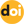The peer-reviewed scientific and technology journal. ISSN: 0204-3327

# Reliability of the RBMK-1000 coolant flow measurement systemhttps://doi.org/10.26583/npe.2018.3.13

### UDC: 62-192:519.6

An analysis of statistical data of diagnostic measurements of two parameters determining the operability of the RBMK-1000 reactor’s SHADR-8A flowmeters – the minimum value of the amplitude negative half-wave of the flow measuring unit input signal and the mean-square deviation over the flowmeter ball rotation period – made it possible to develop a mathematical model of the flow sensor parametric reliability. The mathematical model of the flowmeter reliability is a random process, which is a superposition of two delayed recovery processes. Studying the flow sensor operation reliability model provides an exponential estimate of the probability that the parameters determining the sensor performance will not exceed the specified levels. Using the Bernoulli scheme and the probability-estimating relationship for the sensor performance parameters, it is possible to calculate the probability of failure-free operation of both a single reactor quadrant and the reactor coolant flow measurement system. In addition, it becomes possible to estimate the intensity of the quadrant failures. Important for practice is the ability to predict the number of failed sensors, depending on the time the system is running. The index of the system reliability can be the average number of failed sensors, the ratio for which is given in the paper. All the research results were obtained without any additional assumptions about the laws of random distributions.

The obtained results can easily be generalized to the case when the dimension of the vector of the defining parameters is greater than two. The use of the results of this study is illustrated by calculated quantitative values of the parametric reliability indices of the flow sensors and the reactor coolant flow measurement system.

1. Аugutis Yu., Аlzbutas R., Matuzas V. Reliability control of the SHADR-32 M coolant flow measurement system in the RBMK-1500 reactor. Energetika, 2002, no. 4, pp. 27 -32 (in Russian).
2. Dollezhal’ H.A., Emel’yanov I.YA. Channel Nuclear Power Reactor. Moscow. Atomizdat Publ., 1980, 140 p. (in Russian).
3. Gercbah I.B., Kordonskij H.B. Failure models. Moscow. Sovetskoe Radio Publ., 1966, 166 p. (in Russian).
4. Druzhinin G.V. Reliability of automated systems. Moscow. Energiya Publ., 1977, 534 p. (in Russian).
5. Pronikov A.S. Parametric reliability of machines. Moscow. MGTU im. N.E. Baumana Publ., 2002, 560 p. (in Russian).
6. Rytov S.M. Introduction to Statistical Radiophysics. Part 1. Random Process. Moscow. Nauka, 1976, 494 p. (in Russian).
7. Pugachev V.S., Sinitsyn I.N. The theory of stochastic systems. Moscow. Logos Publ., 2000, 1000 p. (in Russian).
8. Prokhorov Yu.V., Rozanov Yu.А. The theory of stochastic systems. Studies’. benefit. Probability theory. Basic concept. Limit theorem. Random process. Moscow. Nauka Publ., 1987, 494 p. (in Russian).
9. Korolyuk V.S., Portenko N.I., Skorokhod А.V., Turbin А.F. Handbook of probability theory and mathematical statistics. Kiev. Naukova dumka Publ., 1978, 581 p. (in Russian).
10. Gikhman I.I., Skorokhod А.V. Stochastic differential equation. Kiev. Naukova dumka Publ., 1968, 365 p. (in Russian).
11. Аbramov O.V., Katueva Ya.V. Parallel algorithms for analysis and optimization of parametric reliability. Nadezhnost’, 2005, no. 4, pp. 19-26 (in Russian).
12. Pereguda А.I., Аndreev А.G. The asymptotic method of calculation of indicators of durability of the products functioning in the conditions of shock loadings. Nadezhnost’, 2007, no. 3 (22), pp. 31-39 (in Russian).
13. Pereguda А.I., Аndreev А.G. Evaluation of reliability and durability of products exposed to multi-dimensional cyclic effects. Atomnaya energiya, 2007, v. 102, iss. 6, pp. 351-358 (in Russian).
14. Pereguda А.I., Soborova I.А. Reliability and durability. Models, indicators and methods of their calculation. Obninsk. IATE Publ., 2006, 225 p. (in Russian).
15. Pereguda A.I., Belozerev V.I. Prediction of reliability of flow sensors of heat carrier SHADR-32M. Izvestiya vuzov. Yadernaya energetika. 2017, no. 1, pp. 51-62 (in Russian).
16. Bajkhel’t F., Franken P. Reliability and maintenance. Mathematical approach: Moscow. Radio i svyaz’ Publ., 1988, 393 p. (in Russian).
17. Loehv M. Probability theory. Moscow. Izdatel’stvo Inostrannoy Literatyry Publ.,1962, 720 p. (in Russian).